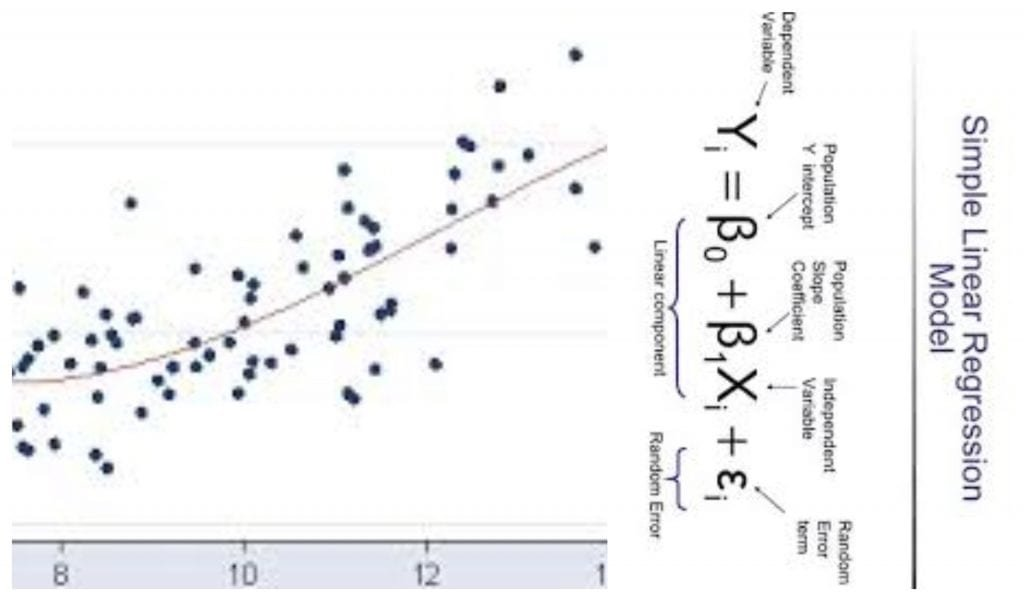Get inspired by the success stories of our students in IIT JAM MS, ISI  MStat, CMI MSc Data Science.  Learn More

# Invariant Regression Coefficient | ISI MStat 2019 PSB Problem 8This is a problem from ISI MStat Examination, 2019. This tests one's familiarity with the simple and multiple linear regression model and estimation of model parameters and is based on the Invariant Regression Coefficient.

## The Problem- Invariant Regression Coefficient

Suppose $\{ (x_i,y_i,z_i):i=1,2,…,n \}$ is a set of trivariate observations on three variables:$X,Y,Z$,, where $z_i=0$ for $i=1,2,…,n-1$ and $z_n=1$.Suppose the least squares linear regression equation of $Y$ on $X$ based on the first $n-1$ observations is $y=\hat{\alpha_0}+\hat{\alpha_1}x$ and the least squares linear regression equation of $Y$ on $X$ and $Z$ based on all the $n$ observations is $y=\hat{\beta_0}+\hat{\beta_1}x+\hat{\beta_2}z$ . Show that $\hat{\alpha_1}=\hat{\beta_1}$.

## Prerequisites

1.Knowing how to estimate the parameters in a linear regression model (Least Square sense)

2. Brief idea about multiple linear regression.

## Solution

Based on the first $n-1$ observations, as $z_i=0$, so, we consider a typical linear regression model of $Y$ on $X$.

Thus,the least square estimate is given by $\hat{\alpha_1}=\frac{\sum_{i=1}^{n-1} (x_i-\bar{x})(y_i-\bar{y})}{\sum_{i=1}^{n-1} (x_i-\bar{x})^2}$

And in the second case, we have:

$y_1=\beta_0+\beta_1 x_1+\epsilon_1$

$y_2=\beta_0+\beta_1 x_2+ \epsilon_2$

$\vdots$

$y_n=\beta_{0}+\beta_1 x_n+\beta_2+ \epsilon_n$

Thus, the error sum of squares for this model is given by:

$SSE=\sum_{i=1}^{n-1} (y_i-\beta_0-\beta_1 x_i)^2+(y_n-\beta_1 x_n -\beta_0 -\beta_2)^2$ , as $z_n=1$.

By differentiating SSE with respect to $\beta_2$, at the optimal value, we must have:

$\hat{\beta}_2 = y_n -\hat{\beta_1}x_n-\hat{\beta_0}$

That is, the last term of SSE must vanish to attain optimality.

So, it is again equivalent to minimize

$\sum_{i=1}^{n-1} (y_i-\beta_0-\beta_1 x_i)^2$ with respect to $\beta_{0} ,\beta_{1}$

This, is nothing but the simple linear regression model again and thus, $\hat{\beta_1}=\hat{\alpha_1}$ and furthermore, $\hat{\beta_0}=\hat{\alpha_0}$.

## Food For Thought

Suppose you have two sets of independent samples. Let they be $\{ (y_1,x_1), ...(y_{n_1},x_{n_1}) \}$ and $\{ (y_{n_1 +1},x_{n_1 +1} ) ,...,(y_{n_1 + n_2} ,x_{n_1 + n_2} ) \}$.

Now you want to fit 2 models to these samples:

$y_i=\beta_0 + \beta_1 x_i + \epsilon_i$ for $i=1,2,..,n_1$

and

$y_i=\gamma_0 + \gamma_1 x_i + \epsilon_i$ for $i=n_1 +1 ,.. ,n_1 + n_2$

Can you write these two models as a single model?

After that ,considering all assumptions for linear regression to be true (If you are not aware of these assumptions you may browse through any regression book or search the internet), is it justifiable to infer $\beta_1 = \gamma_1$ ?

This is a problem from ISI MStat Examination, 2019. This tests one's familiarity with the simple and multiple linear regression model and estimation of model parameters and is based on the Invariant Regression Coefficient.

## The Problem- Invariant Regression Coefficient

Suppose $\{ (x_i,y_i,z_i):i=1,2,…,n \}$ is a set of trivariate observations on three variables:$X,Y,Z$,, where $z_i=0$ for $i=1,2,…,n-1$ and $z_n=1$.Suppose the least squares linear regression equation of $Y$ on $X$ based on the first $n-1$ observations is $y=\hat{\alpha_0}+\hat{\alpha_1}x$ and the least squares linear regression equation of $Y$ on $X$ and $Z$ based on all the $n$ observations is $y=\hat{\beta_0}+\hat{\beta_1}x+\hat{\beta_2}z$ . Show that $\hat{\alpha_1}=\hat{\beta_1}$.

## Prerequisites

1.Knowing how to estimate the parameters in a linear regression model (Least Square sense)

2. Brief idea about multiple linear regression.

## Solution

Based on the first $n-1$ observations, as $z_i=0$, so, we consider a typical linear regression model of $Y$ on $X$.

Thus,the least square estimate is given by $\hat{\alpha_1}=\frac{\sum_{i=1}^{n-1} (x_i-\bar{x})(y_i-\bar{y})}{\sum_{i=1}^{n-1} (x_i-\bar{x})^2}$

And in the second case, we have:

$y_1=\beta_0+\beta_1 x_1+\epsilon_1$

$y_2=\beta_0+\beta_1 x_2+ \epsilon_2$

$\vdots$

$y_n=\beta_{0}+\beta_1 x_n+\beta_2+ \epsilon_n$

Thus, the error sum of squares for this model is given by:

$SSE=\sum_{i=1}^{n-1} (y_i-\beta_0-\beta_1 x_i)^2+(y_n-\beta_1 x_n -\beta_0 -\beta_2)^2$ , as $z_n=1$.

By differentiating SSE with respect to $\beta_2$, at the optimal value, we must have:

$\hat{\beta}_2 = y_n -\hat{\beta_1}x_n-\hat{\beta_0}$

That is, the last term of SSE must vanish to attain optimality.

So, it is again equivalent to minimize

$\sum_{i=1}^{n-1} (y_i-\beta_0-\beta_1 x_i)^2$ with respect to $\beta_{0} ,\beta_{1}$

This, is nothing but the simple linear regression model again and thus, $\hat{\beta_1}=\hat{\alpha_1}$ and furthermore, $\hat{\beta_0}=\hat{\alpha_0}$.

## Food For Thought

Suppose you have two sets of independent samples. Let they be $\{ (y_1,x_1), ...(y_{n_1},x_{n_1}) \}$ and $\{ (y_{n_1 +1},x_{n_1 +1} ) ,...,(y_{n_1 + n_2} ,x_{n_1 + n_2} ) \}$.

Now you want to fit 2 models to these samples:

$y_i=\beta_0 + \beta_1 x_i + \epsilon_i$ for $i=1,2,..,n_1$

and

$y_i=\gamma_0 + \gamma_1 x_i + \epsilon_i$ for $i=n_1 +1 ,.. ,n_1 + n_2$

Can you write these two models as a single model?

After that ,considering all assumptions for linear regression to be true (If you are not aware of these assumptions you may browse through any regression book or search the internet), is it justifiable to infer $\beta_1 = \gamma_1$ ?

This site uses Akismet to reduce spam. Learn how your comment data is processed.

### Knowledge Partner